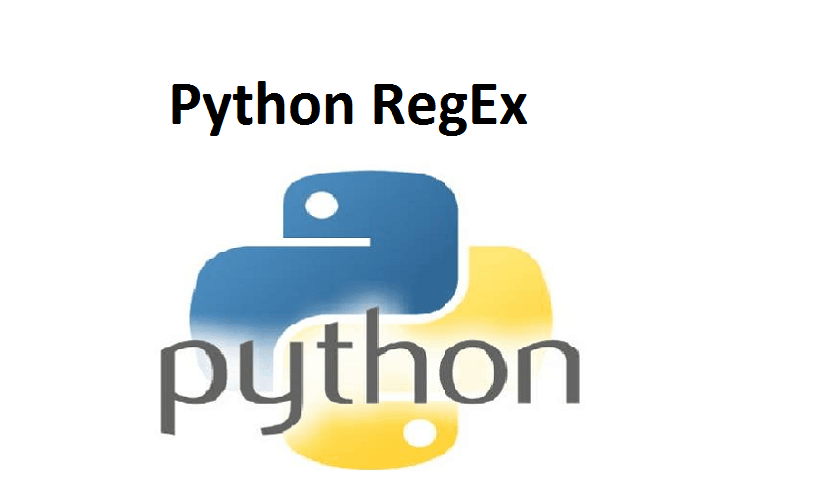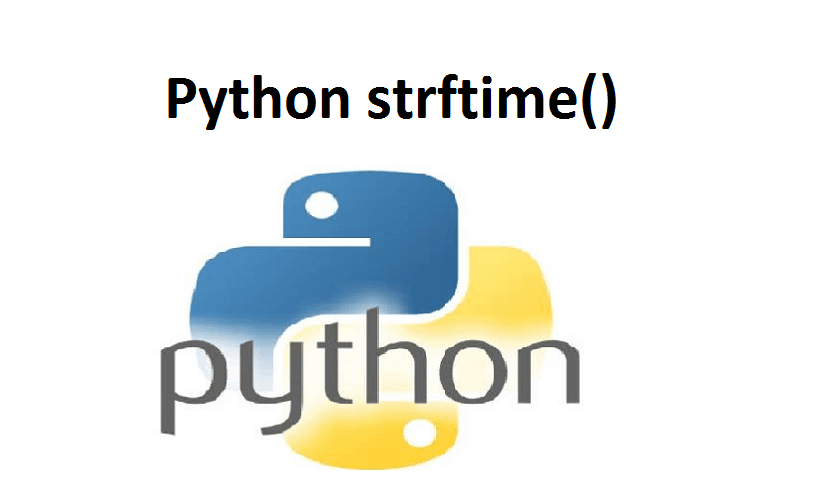# Python datetimePython datetime: In this article, you will learn how to manipulate date and time in Python with the help of examples.

Python has a module named datetime to work with dates and times. let’s create a few simple programs related to date and time before we dig deeper.

Contents

## Example 1: Get Current Date and Time

```import datetime

datetime_object = datetime.datetime.now()
print(datetime_object)```

When you run the program, the output will be something like:

`2020-6-22 01:51:03.478039`

Here, we have imported datetime module using an import datetime statement.

One of the classes defined in the datetime module is datetime class. We then used now() method to create a datetime object containing the current local date and time.

## Example 2: Get Current Date

```import datetime

date_object = datetime.date.today()
print(date_object)```

When you run the program, the output will be something like:

```2020-6-22
In this program, we have used today() method defined in the date class to get a
date object containing the current local date.
```

## What’s inside DateTime?

We can use the dir() function to get a list containing all attributes of a module.

```import datetime

print(dir(datetime))```

When you run the program, the output will be:

```
['MAXYEAR', 'MINYEAR', '__builtins__', '__cached__', '__doc__', '__file__', '__loader__', '__name__', '__package__', '__spec__', '_divide_and_round', 'date', 'datetime', 'datetime_CAPI', 'time', 'timedelta', 'timezone', 'tzinfo']```

Commonly used classes in the datetime module are:

date Class
time Class
datetime Class
timedelta Class

## DateTime.date Class

You can instantiate data objects from the date class. A date object represents a date (year, month, and day).

## Example 3: Date object to represent a date

```import datetime

d = datetime.date(2020, 6, 22)
print(d)```

When you run the program, the output will be:

`2020-06-22`

If you are wondering, date() in the above example is a constructor of the date class. The constructor takes three arguments: year, month, and day.

A variable is a date object.

We can only import the date class from the DateTime module. Here’s how:

```from datetime import date

a = date(2020, 6, 22)
print(a)```

## Example 4: Get current date

You can create a date object containing the current date by using a class method named today(). Here’s how:

```from datetime import date

today = date.today()

print("Current date =", today)```

## Example 5: Get date from a timestamp

We can also make date objects from a timestamp. A Unix timestamp is the number of seconds between a specific date and January 1, 1970, at UTC. You can change over a timestamp to date using from timestamp() strategy.

```from datetime import date

timestamp = date.fromtimestamp(1326244364)
print("Date =", timestamp)```

When you run the program, the output will be:

```Date = 2012-01-11
```

## Example 6: Print today’s year, month and day

We can get the year, month, day, day of the week, etc. from the date object easily. Here’s how:

```from datetime import date

# date object of today's date
today = date.today()

print("Current year:", today.year)
print("Current month:", today.month)
print("Current day:", today.day)```

## DateTime.time

A time object instantiated from the time class represents the local time.

## Example 7: Time object to represent time

```from datetime import time

# time(hour = 0, minute = 0, second = 0)
a = time()
print("a =", a)

# time(hour, minute and second)
b = time(11, 34, 56)
print("b =", b)

# time(hour, minute and second)
c = time(hour = 11, minute = 34, second = 56)
print("c =", c)

# time(hour, minute, second, microsecond)
d = time(11, 34, 56, 234566)
print("d =", d)```

When you run the program, the output will be:

```
a = 00:00:00
b = 11:34:56
c = 11:34:56
d = 11:34:56.234566```

## Example 8: Print hour, minute, second and microsecond

Once you create a time object, you can easily print its attributes such as hour, minute, etc.

```from datetime import time

a = time(11, 34, 56)

print("hour =", a.hour)
print("minute =", a.minute)
print("second =", a.second)
print("microsecond =", a.microsecond)```

When you run the example, the output will be:

```
hour = 11
minute = 34
second = 56
microsecond = 0```

Notice that we haven’t passed a microsecond argument. Hence, its default value 0 is printed.

## datetime.datetime

The DateTime module has a class named date class that can contain information from both date and time objects.

## Example 9: Python DateTime object

```from datetime import datetime

#datetime(year, month, day)
a = datetime(2018, 11, 28)
print(a)

# datetime(year, month, day, hour, minute, second, microsecond)
b = datetime(2017, 11, 28, 23, 55, 59, 342380)
print(b)
```

When you run the program, the output will be:

```2018-11-28 00:00:00
2017-11-28 23:55:59.342380```

The first three arguments year, month, and day in the DateTime() constructor are mandatory.

## Example 10: Print year, month, hour, minute and timestamp

```from datetime import datetime

a = datetime(2017, 11, 28, 23, 55, 59, 342380)
print("year =", a.year)
print("month =", a.month)
print("hour =", a.hour)
print("minute =", a.minute)
print("timestamp =", a.timestamp())```

When you run the program, the output will be:

```
year = 2017
month = 11
day = 28
hour = 23
minute = 55
timestamp = 1511913359.34238```

## DateTime.timedelta

A time delta object represents the difference between two dates or times.

## Example 11: Difference between two dates and times

```from datetime import datetime, date

t1 = date(year = 2018, month = 7, day = 12)
t2 = date(year = 2017, month = 12, day = 23)
t3 = t1 - t2
print("t3 =", t3)

t4 = datetime(year = 2018, month = 7, day = 12, hour = 7, minute = 9, second = 33)
t5 = datetime(year = 2019, month = 6, day = 10, hour = 5, minute = 55, second = 13)
t6 = t4 - t5
print("t6 =", t6)

print("type of t3 =", type(t3))
print("type of t6 =", type(t6))  ```

When you run the program, the output will be:

```
t3 = 201 days, 0:00:00
t6 = -333 days, 1:14:20
type of t3 = <class 'datetime.timedelta'>
type of t6 = <class 'datetime.timedelta'>```

Notice, both t3 and t6 are of <class ‘DateTime.timedelta’> type.

## Example 12: Difference between two-time delta objects

```from datetime import timedelta

t1 = timedelta(weeks = 2, days = 5, hours = 1, seconds = 33)
t2 = timedelta(days = 4, hours = 11, minutes = 4, seconds = 54)
t3 = t1 - t2

print("t3 =", t3)```

When you run the program, the output will be:

```
t3 = 14 days, 13:55:39```

Here, we have created two-time delta objects t1 and t2, and their difference is printed on the screen.

## Example 13: Printing negative timedelta object

```from datetime import timedelta

t1 = timedelta(seconds = 33)
t2 = timedelta(seconds = 54)
t3 = t1 - t2

print("t3 =", t3)
print("t3 =", abs(t3))```

When you run the program, the output will be:

```t3 = -1 day, 23:59:39
t3 = 0:00:21

```

## Example 14: Time duration in seconds

You can get the total number of seconds in a time delta object using the total_seconds() method.

```from datetime import timedelta

t = timedelta(days = 5, hours = 1, seconds = 33, microseconds = 233423)
print("total seconds =", t.total_seconds())```

When you run the program, the output will be:

`total seconds = 435633.233423`

You can also find sum of two dates and times using + operator. Also, you can multiply and divide a time delta object by integers and floats.

## Python format DateTime

The way date and time are represented may be different in different places, organizations, etc. It’s more common to use mm/dd/yyyy in the US, whereas dd/mm/yyyy is more common in the UK.

Python has strftime() and strptime() methods to handle this.

## Python strftime() – datetime object to string

The strftime() method is defined under classes date, DateTime, and time. The method creates a formatted string from a given date, DateTime, or time object.

## Example 15: Format date using strftime()

```from datetime import datetime

# current date and time
now = datetime.now()

t = now.strftime("%H:%M:%S")
print("time:", t)

s1 = now.strftime("%m/%d/%Y, %H:%M:%S")
# mm/dd/YY H:M:S format
print("s1:", s1)

s2 = now.strftime("%d/%m/%Y, %H:%M:%S")
# dd/mm/YY H:M:S format
print("s2:", s2)```

When you run the program, the output will be something like:

```
time: 04:34:52
s1: 12/26/2018, 04:34:52
s2: 26/12/2018, 04:34:52```

Here, %Y, %m, %d, %H, etc. are format codes. The strftime() method takes one or more format codes and returns a formatted string based on it.

In the above program, t, s1, and s2 are strings.

%Y – year [0001,…, 2018, 2019,…, 9999]
%m – month [01, 02, …, 11, 12]
%d – day [01, 02, …, 30, 31]
%H – hour [00, 01, …, 22, 23
%M – minute [00, 01, …, 58, 59]
%S – second [00, 01, …, 58, 59]

## Python strptime() – string to datetime

The strptime() method creates a DateTime object from a given string (representing date and time).

## Example 16: strptime()

```from datetime import datetime

date_string = "21 June, 2018"
print("date_string =", date_string)

date_object = datetime.strptime(date_string, "%d %B, %Y")
print("date_object =", date_object)```

When you run the program, the output will be:

```
date_string = 21 June, 2018
date_object = 2018-06-21 00:00:00```

The strptime() method takes two arguments:

a string representing date and time
format code equivalent to the first argument
By the way, %d, %B, and %Y format codes are used for the day, month(full name), and a year respectively.

## Handling of timezone in Python

Assume, you are working on a project and need to show date and time-dependent on their timezone. Instead of attempting to deal with the timezone yourself, use a third-party pytZ module.

```from datetime import datetime
import pytz

local = datetime.now()
print("Local:", local.strftime("%m/%d/%Y, %H:%M:%S"))

tz_NY = pytz.timezone('America/New_York')
datetime_NY = datetime.now(tz_NY)
print("NY:", datetime_NY.strftime("%m/%d/%Y, %H:%M:%S"))

tz_London = pytz.timezone('Europe/London')
datetime_London = datetime.now(tz_London)
print("London:", datetime_London.strftime("%m/%d/%Y, %H:%M:%S"))```

When you run the program, the output will be something like:

```Local time: 2018-12-20 13:10:44.260462
America/New_York time: 2018-12-20 13:10:44.260462
Europe/London time: 2018-12-20 13:10:44.260462	```

Here, datetime_NY and datetime_London are DateTime objects containing the current date and time of their respective timezone.

Please feel free to give your comment if you face any difficulty here.

For more Articles click on the below link.### Python RegEx### Python strftime()